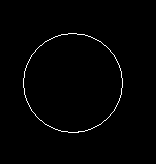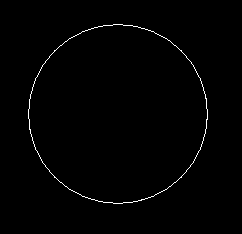# Draw circle in C graphics

• Difficulty Level : Easy
• Last Updated : 06 Dec, 2019

The header file graphics.h contains circle() function which draws a circle with center at (x, y) and given radius.

Syntax :

Take a step-up from those "Hello World" programs. Learn to implement data structures like Heap, Stacks, Linked List and many more! Check out our Data Structures in C course to start learning today.
```circle(x, y, radius);

where,
(x, y) is center of the circle.
```

Examples :

```Input : x = 250, y = 200, radius = 50
Output :Input : x = 300, y = 150, radius = 90
Output :```

Below is the implementation to draw circle in C:

 `// C Implementation for drawing circle``#include `` ` `//driver code``int` `main()``{``    ``// gm is Graphics mode which is``    ``// a computer display mode that``    ``// generates image using pixels.``    ``// DETECT is a macro defined in``    ``// "graphics.h" header file``    ``int` `gd = DETECT, gm;`` ` `    ``// initgraph initializes the``    ``// graphics system by loading a``    ``// graphics driver from disk``    ``initgraph(&gd, &gm, ``""``);`` ` `    ``// circle function``    ``circle(250, 200, 50);`` ` `    ``getch();`` ` `    ``// closegraph function closes the``    ``// graphics mode and deallocates``    ``// all memory allocated by``    ``// graphics system .``    ``closegraph();`` ` `    ``return` `0;``}`

Output :

``````
My Personal Notes arrow_drop_up